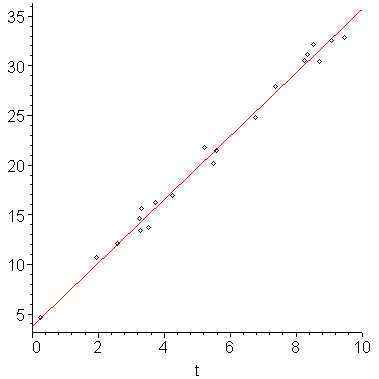## 6 Least Squares Curve Fitting

Given a set of points (xiyi) for i = 0, 1, 2, ..., n, we may not be able (or may not want) to find a function which passes through all points, but rather, we may want to find a function of a particular form which passes as closely as possible to the points. For example, in Figure 1, it would make much more sense to try to find the straight line which passes as closely as possible to each of the points.Figure 1. Linear regression of a straight line on a set of points.

We will look at three techniques for finding functions which are closest to a given curve:

1. Linear regression using linear polynomials (matching straight lines),
2. General linear regression (polynomials, etc.), and
3. Transformations to linear regression (for matching exponential functions).

As well, we will discuss how we can use regression curves for extrapolation and an efficient method (QR decomposition) for calculating least squares curves.

# Terminology

This processes is called regression because the y values are regressing (or moving towards) the value on the curve which we find.

The term linear in linear regression refers to the coefficients of the matching function. As a special case, we begin by looking at linear regression using linear polynomials (i.e., y = ax + b).